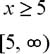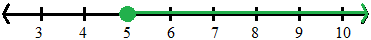Linear Inequalities

Linear Inequalities

Solving a linear inequality is similar to solving a linear equation. The only difference is that when you multiply or divide both sides by a negative number, you must change the direction of the inequality.

6 > 4
-1(6) < -1(4)
-6 < -4

To graph an inequality, mark its position and direction on a number line.

x < 3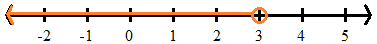The open circle indicates that the value of 3 is not part of the inequality.

x < 3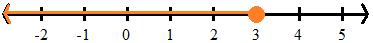The closed circle now indicates that the value of 3 is part of the inequality.

To express an inequality in interval notation:

• Include the span of numbers included in the group from left to right, separated by a comma.
• Use parenthesis next to a number that is excluded from the group. Use a bracket when the number is included in the group.
• Use the infinity or negative infinity symbols to denote when the group goes on indefinitely.  Always use round parentheses symbols with infinity.

x < 3

(-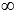, 3)

x < 3

(-, 3]

Example

7 - 4x < -13

As you would with an equation, add or subtract terms to get all variables on one side and all constants on the other.

7 - 4x - 7 < -13 - 7

-4x < -20

Now divide both sides by the coefficient of x.  (Careful, the coefficient in this example is negative!)

-4x / -4 > -20 / -4